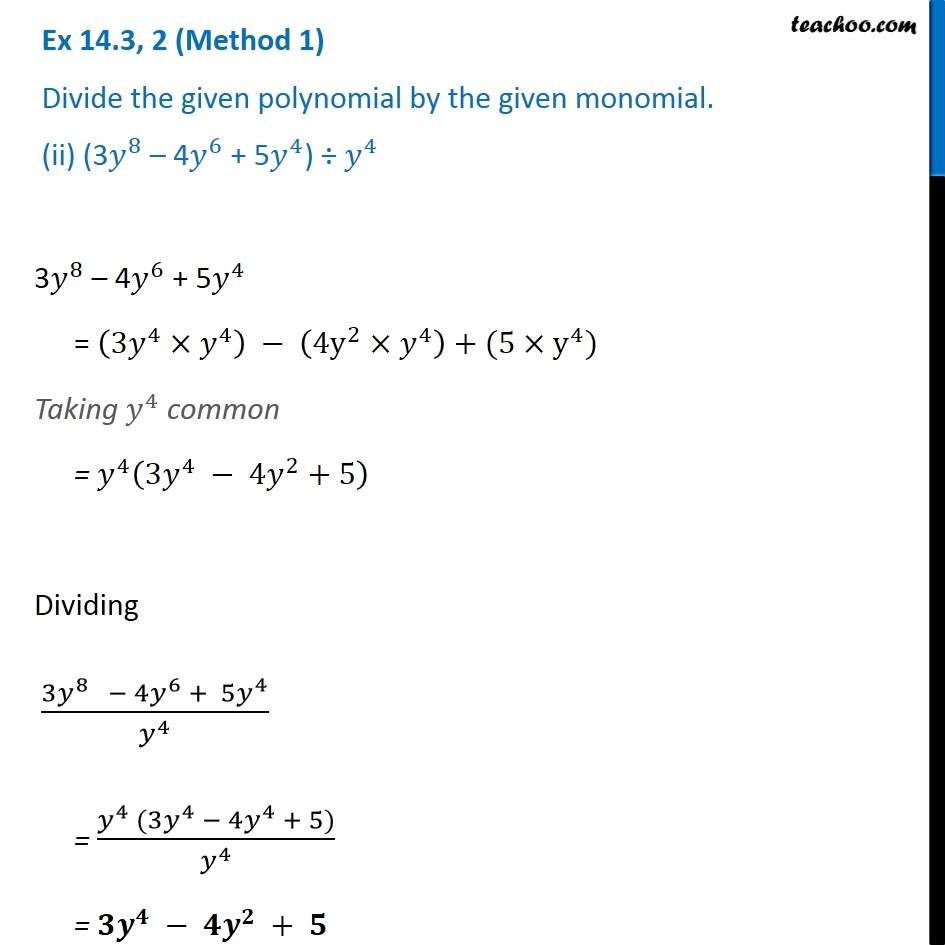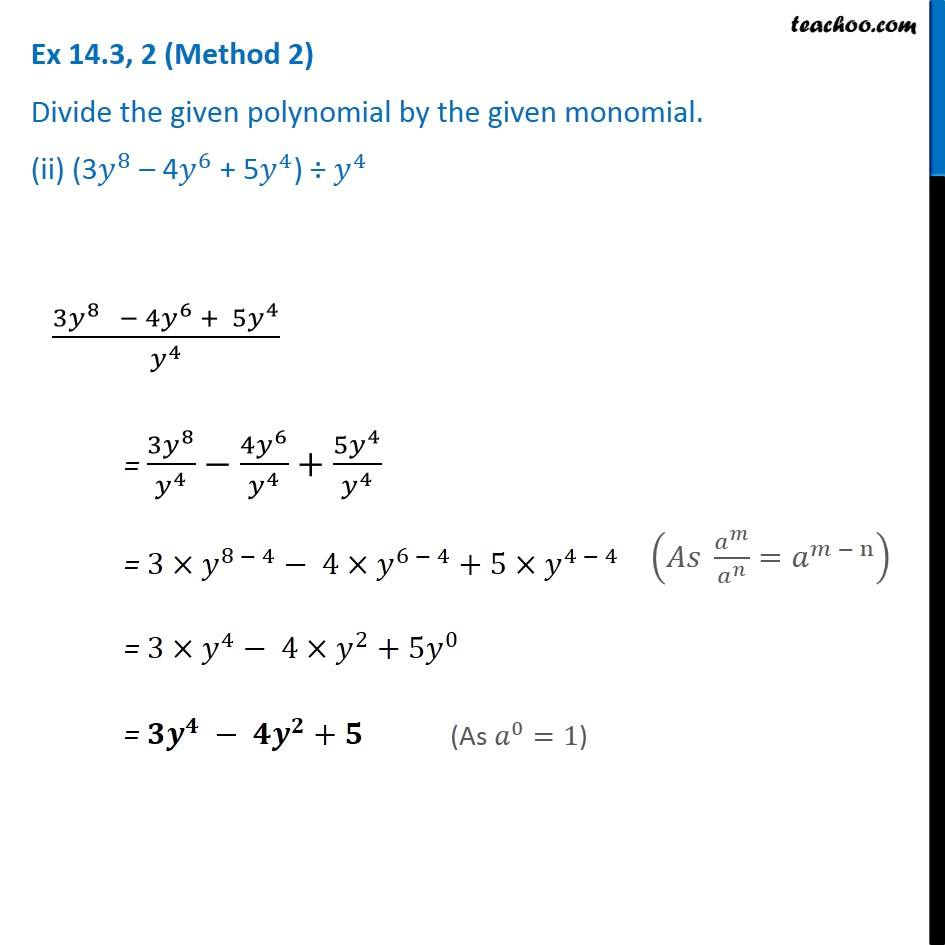Ex 14.3

Chapter 14 Class 8 Factorisation
Serial order wiseGet live Maths 1-on-1 Classs - Class 6 to 12

### Transcript

Ex 14.3, 2 (Method 1) Divide the given polynomial by the given monomial. (ii) (3𝑦^8 – 4𝑦^6 + 5𝑦^4) ÷ 𝑦^4 3𝑦^8 – 4𝑦^6 + 5𝑦^4 = (3𝑦^4×𝑦^4 ) − (4y^2×𝑦^4 )+(5×y^4) Taking 𝑦^4 common = 𝑦^4 (3𝑦^4 − 4𝑦^2+5) Dividing (3𝑦^8 − 4𝑦^6 + 5𝑦^4)/𝑦^4 = (𝑦^4 (3𝑦^4 − 〖4𝑦〗^4 + 5))/𝑦^4 = 𝟑𝒚^𝟒 − 𝟒𝒚^𝟐 + 𝟓 Ex 14.3, 2 (Method 2) Divide the given polynomial by the given monomial. (ii) (3𝑦^8 – 4𝑦^6 + 5𝑦^4) ÷ 𝑦^4 (3𝑦^8 − 4𝑦^6 + 5𝑦^4)/𝑦^4 = (3𝑦^8)/𝑦^4 −〖4𝑦〗^6/𝑦^4 +(5𝑦^4)/𝑦^4 = 3×𝑦^(8 − 4)− 4× 𝑦^(6 − 4)+5×𝑦^(4 − 4) = 3×𝑦^4− 4× 𝑦^2+〖5𝑦〗^0 = 〖𝟑𝒚〗^𝟒 − 𝟒𝒚^𝟐+𝟓 (𝐴𝑠 𝑎^𝑚/𝑎^𝑛 =𝑎^(𝑚 − n) ) (As 𝑎^0=1)